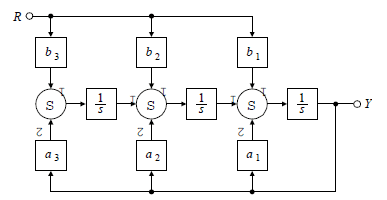# Block diagram simplication

I am having trouble trying to obtain Y/R from the block diagram below:the only thing i understand so far is that the bottom right loop is a feedback loop and can be simplified to 1/(s+a1). i know b1s can be obtained from the right loop, but i am having trouble getting to that point. i know it has to do with moving the summer

## Answers and Replies

I like Serena
Homework Helper
Hi magnifik!Trace the signals R and Y and construct an equation from them.

For instance, the left summation sums b3R and a3Y, which is then multiplied by 1/s.
This yields:
$$\frac 1 s (b_3 R + a_3 Y)$$

Can you complete the expression tracing it up to the right side, where it must be equal to Y?